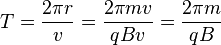# Cyclotron facts for kids

Kids Encyclopedia Facts

A cyclotron is a type of particle accelerator invented by Ernest Lawrence from the University of California, Berkeley in 1930. It accelerates charged particles by whirling them in a circle. The first cyclotrons were the size of a hand. Some modern circular accelerators use a circle as wide as a city.

Cyclotrons use perpendicular magnetic field to bend electrons and particles through into a semicircular path by an applied electric field. The applied electric field accelerates electrons between the "D" electrodes(also called "dees") of the magnetic field region.

The accelerating electric field reverses just at the time the electrons finish their half circle, so that it accelerates them across the gap. With a higher speed, they move in a larger semicircle. After repeating this process several times, they come out the exit port at a high speed.

## Cyclotron Frequency

A moving charge in a cyclotron will move in a circular path under the influence of a constant magnetic field. If the time to complete one orbit is calculated:$T=\frac{2\pi r}{v}=\frac{2\pi mv}{qBv}=\frac{2\pi m}{qB}$.

It is found that the period is independent of the radius. Therefore if a square wave is applied at angular frequency qB/m, the charge will spiral outward, increasing in speed.

When a square wave of angular frequency ω=qB/m is applied between the two sides of the magnetic poles, the charge will be boosted again at just the right time to accelerate it across the gap. Thus the constant cyclotron frequency can continue to accelerate the charge (so long as it is not relativistic).

## Images for kidsCyclotron Facts for Kids. Kiddle Encyclopedia.